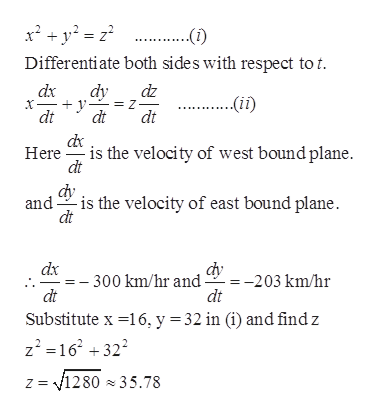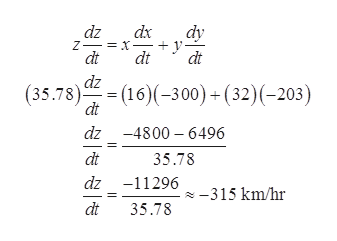Question
60 views

# One airplane is approaching an airport from the north at 203 km/hr. a second airplane approaches from the east at 300 km/hr. find the rate at which the distance between the planes changes when the southbound plane is 32 km away from the airport and the westbound plane is 16 km from the airport.

check_circle

Step 1

Let in the below figure P represents the airport, Q represents the southbound plane and R represents the westbound plane.

Then x = 16 km, y = 32 km

Step 2

Using Pythagoras theorem in triangle PQRhelp_outlineImage Transcriptionclosex2y2 Differentiate both sides with respect to t dy = Z dt dx dz --(ii) dt dt Hereis the velocity of west bound plane dt andis the velocity of east bound plane. dx = -203 km/hr dt - 300 km/hr and dt Substitute x 16, y = 32 in (i) and find z z2=16 +32 = 1280 x 35.78 fullscreen
Step 3

Now find the change of distance between two planes b...help_outlineImage Transcriptionclosedz dx = x- dt dy dt dt dz (35.78)(16)(-300)(32)(-203) dt dz 4800 6496 dt 35.78 dz-11296 315 km/hr dt 35.78 fullscreen

### Want to see the full answer?

See Solution

#### Want to see this answer and more?

Solutions are written by subject experts who are available 24/7. Questions are typically answered within 1 hour.*

See Solution
*Response times may vary by subject and question.
Tagged in

### Derivative